CONTRAST Statement
CONTRAST ’label’ effect values <...effect values> </ options> ;

The CONTRAST statement enables you to define custom hypothesis tests by specifying anvector or matrix for testing the hypothesis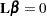. Thus, to use this feature you must be familiar with the details of the model parameterization used in PROC GLM. For more information, see the section Parameterization of PROC GLM Models of Chapter 41, The GLM Procedure. All of the elements of thevector can be given, or if only certain portions of thevector are given, the remaining elements are constructed by PROC GLMPOWER from the context (in a manner similar to rule 4 discussed in the section Construction of Least Squares Means of Chapter 41, The GLM Procedure ).

There is no limit to the number of CONTRAST statements you can specify. Each sample size analysis includes tests for all CONTRAST statements.

In the CONTRAST statement,

label

identifies the contrast on the output. A label is required for every contrast specified. Labels must be enclosed in quotes.

effect

identifies an effect that appears in the MODEL statement, or the INTERCEPT effect. You do not need to include all effects that are in the MODEL statement.

values

are constants that are elements of thevector associated with the effect.

You can specify the following option in the CONTRAST statement after a slash (/):

SINGULAR=number

tunes the estimability checking. If ABS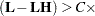number for any row in the contrast, thenis declared nonestimable.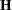is the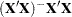matrix, and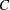is ABS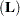except for rows whereis zero, and then it is 1. The default value for the SINGULAR= option is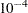. Values for the SINGULAR= option must be between 0 and 1.

The CONTRAST statement enables you to perform custom hypothesis tests. If the hypothesis is estimable, then the sum of squares due to it, SS(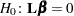), is computed as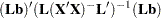where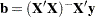is the estimated solution vector.

The degrees of freedom associated with the hypothesis are equal to the row rank of. The sum of squares computed in this situation is equivalent to the sum of squares computed using anmatrix with any row deleted that is a linear combination of previous rows.

Multiple-degrees-of-freedom hypotheses can be specified by separating the rows of thematrix with commas.